• 官方微信• 客服微信• 在线报名
• XML地图
• TAGS

### 乐知舟儿童教育：一年级数学加减口诀表+口算技巧+口算练习，10分钟教20以内进位加法

（掌握“凑十法”，提倡“递推法”。）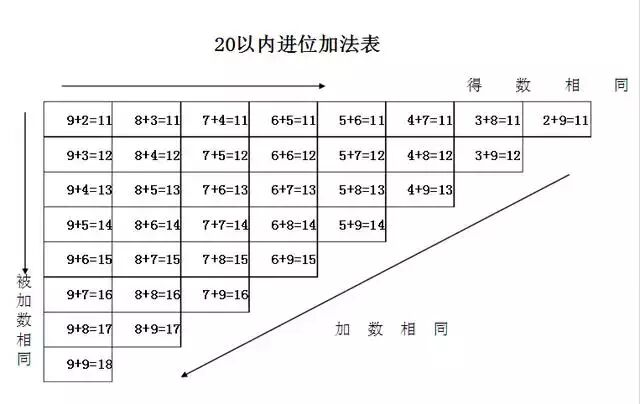20以内退位减法

20以内退位减，口算方法和简单。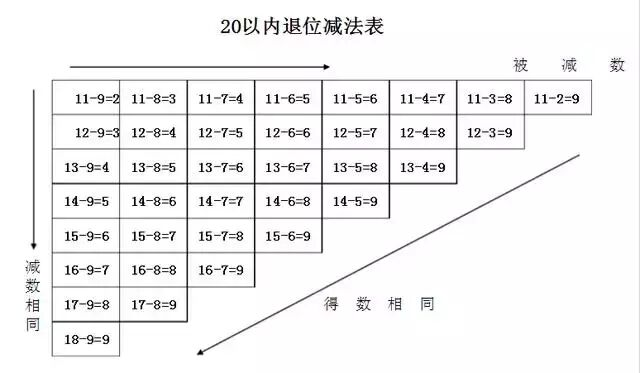10以内加法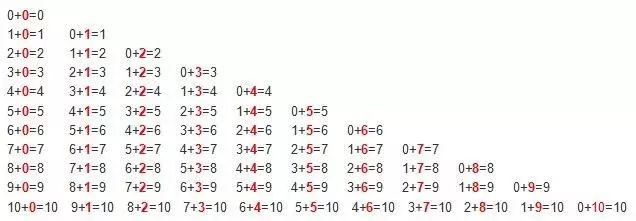10以内减法口诀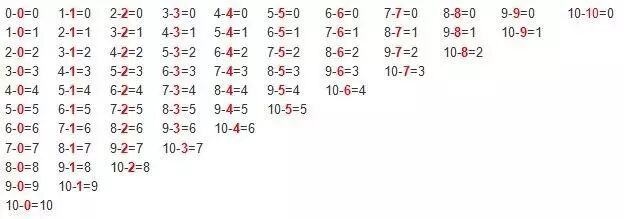20以内的进位加法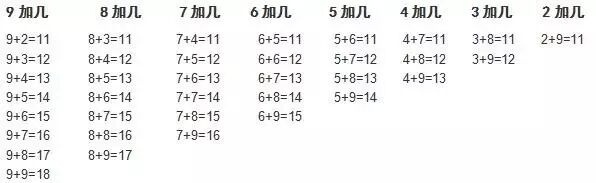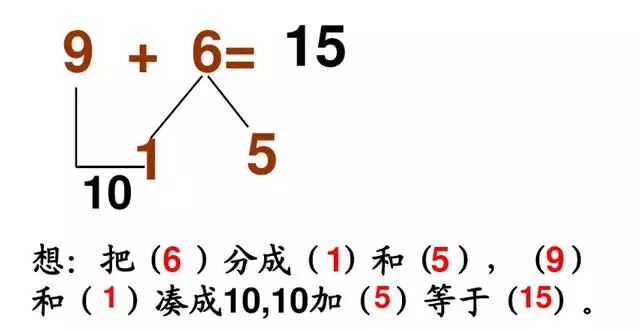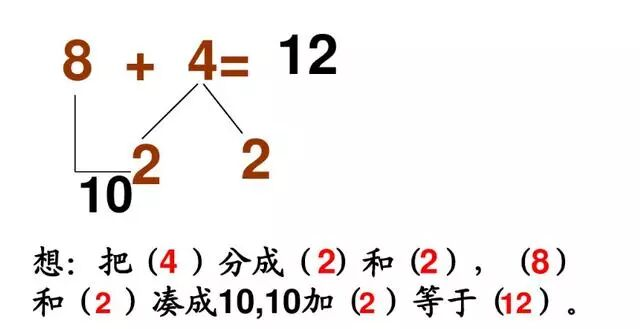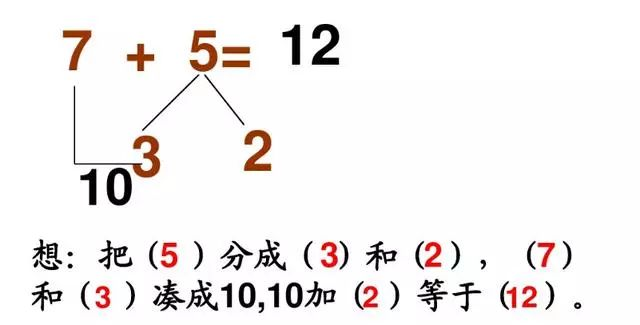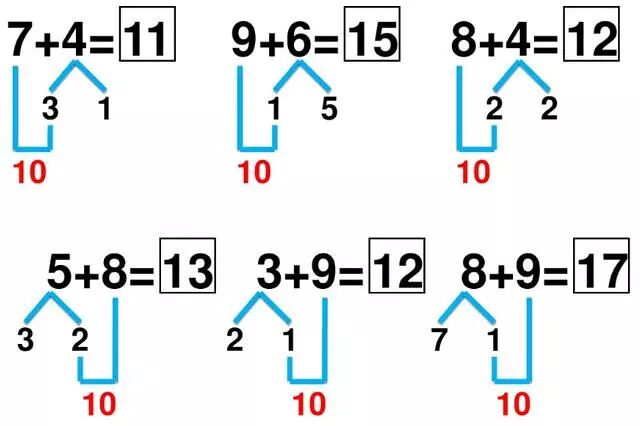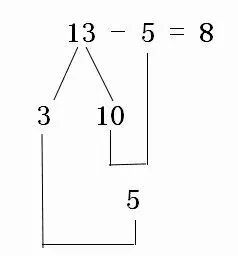15-8=？为例，可以将其拆成连减法来计算，15先减去5，再减去3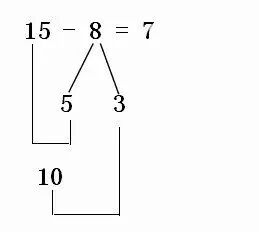2+2=    5+4=     3+0=      0+4=     0+0=

3+0=    3+1=      5+4=     0+4=     0+1=

0+1=     0+2=     3+1=     0+1=     0+4=

4-3=      5-4=      2+1=     1-0=      5-4=

4-1=      3-3=       4-3=      2-0=      0-0=

2+2=     5+4=      0+1=     3+3=     2+5=

4+4=     3+3=      2+2=     3+1=     1+0=

3+4=     3+5=      4+2=      2+1=    2+7=

4-3=       1-1=      5-4=       3-3=      4-3=

5-2=       4-3=       5-5=      5-0=      1-0=

5-4=    4-2=     4-0=     3-3=     5-3=

3+1=    2+1=    0+3=     0+5=     4+4=

2+2=    4+4=    1+4=    3+2=     2+1=

4-1=    5-2=    5-5=     3-0=     5-2=

3-0=    4-2=    3-2=     2-2=     3-0=

5+3=    0+2=    4+4=    1+3=     1+3=

5-2=     3-3=     4-2=     1-1=      3-1=

4+3=    4+4=    5+3=    1+2=     2+4=

5-5=    4+2=     2+1=      5+2=     2+3=

5-4=    5-5=    4-2=    5-1=     1-1=

4+0=    0+3=   0+3=   5+3=    2+3=

4+3=    2+1=   4+4=   5+1=    2+4=

3-1=    5-3=    4-1=     3-0=    5-2=

5-3=    3-1=    5-4=    4-0=    3-0=

4-4=    4-1=    5-3=    2-1=    3-1=

3+4=    5+5=    4+4=    0+1=   3+4=

2+1=    3+2=    0+3=    4+2=    2+3=

3+4=    4+1=    1+5=    2+4=    0+3=

2-2=    1-0=     4-2=      5-3=    2-0=

5-5=    3-1=     4-3=      5-3=    3-0=

5-2=    3-3=    4-2=     1-1=     3-1=

4+3=    4+4=    5+3=    1+2=    2+4=

5-5=    4+2=    2+1=      5+2=    2+3=

5-4=    5-5=    4-2=    5-1=    1-1=

5-4=    4-2=    4-0=    3-3=    5-3=

3+1=    2+1=    0+3=     0+5=    4+4=

2+2=    4+4=    1+4=    3+2=    2+1=

4-1=    5-2=    5-5=     3-0=    5-2=

3-0=    4-2=    3-2=     2-2=    3-0=

5+3=    0+2=    4+4=    1+3=     1+3=

▼▼

▼▼▼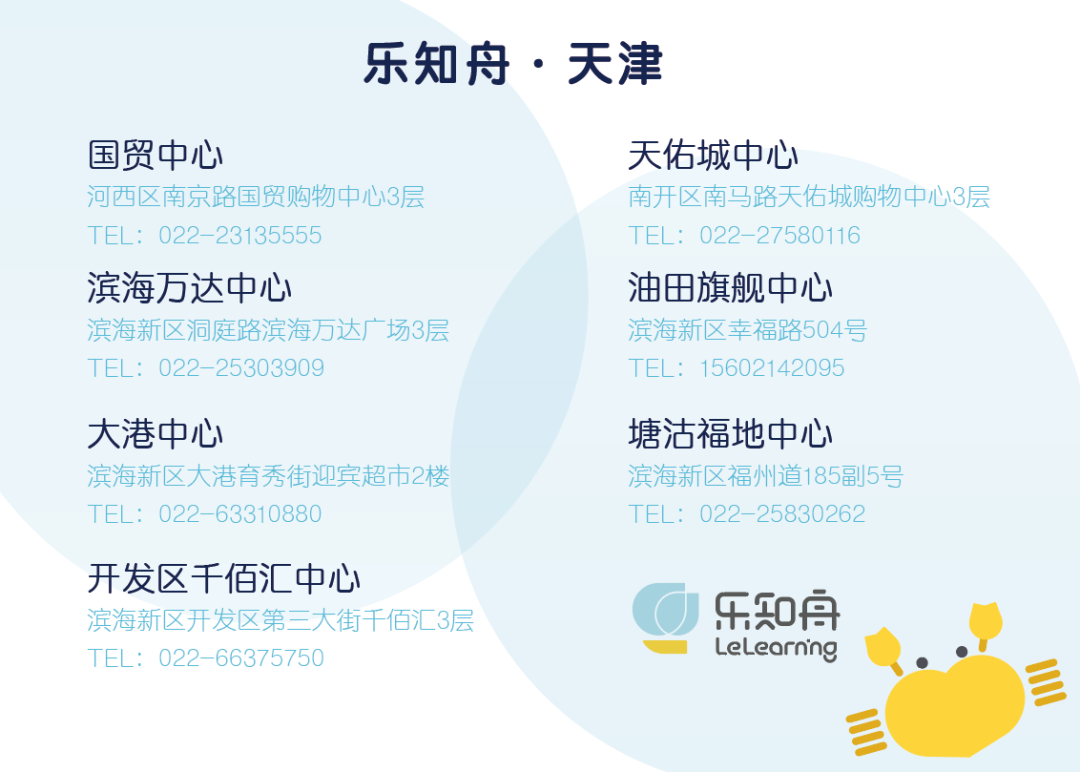400-6161-555